FTSE - 2D research
A Mars heliocentric planetary fan set at 2 points per 1 degree of Mars travelled, from the Low of July 12th 1984 ,gives the Dec 30 1999
Top

978 to 6950 =5972 points and Mars Travelled 2974.212 degrees

So  2974.212 x  2 + 978.7 =6927.124

Actual
High was 6950 .6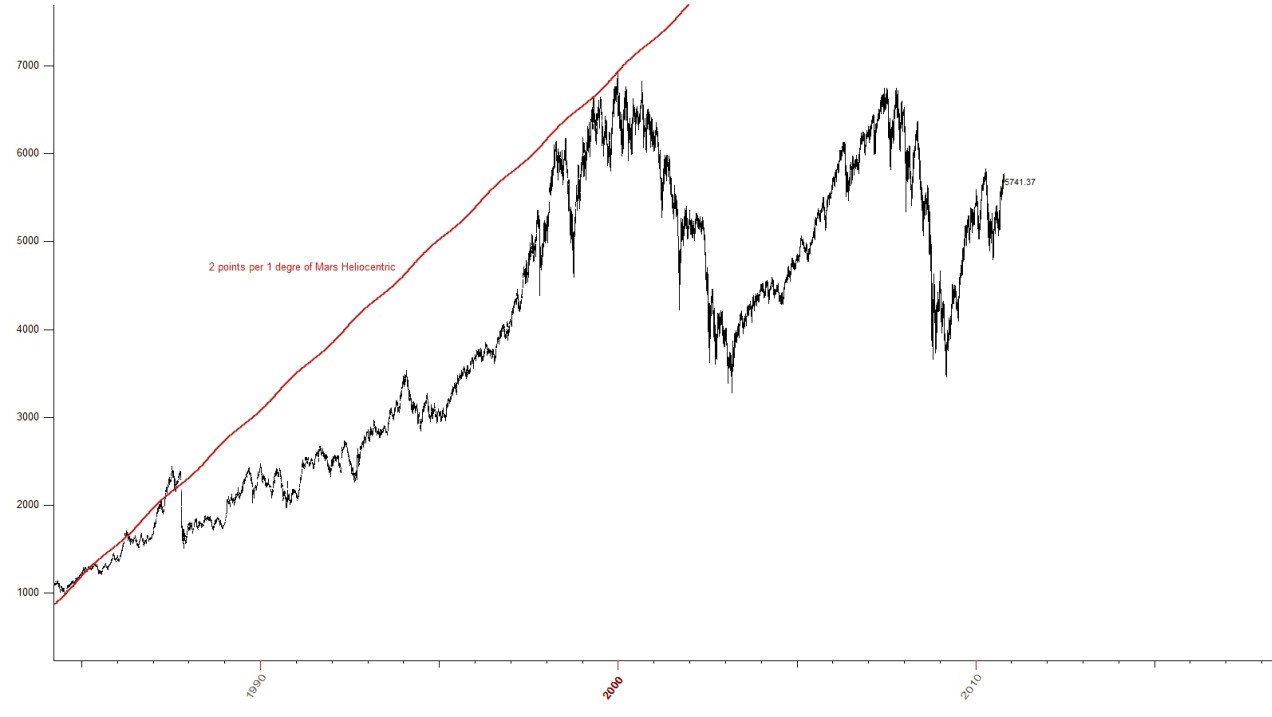Again a Mars Heliocentric planetary Fan set at 2 points per 1 degree travelled ,this time from the Dec 30th 1999 Top ,gives the March 9th 2009 LOWHere are both Mars Heliocentric planetary fans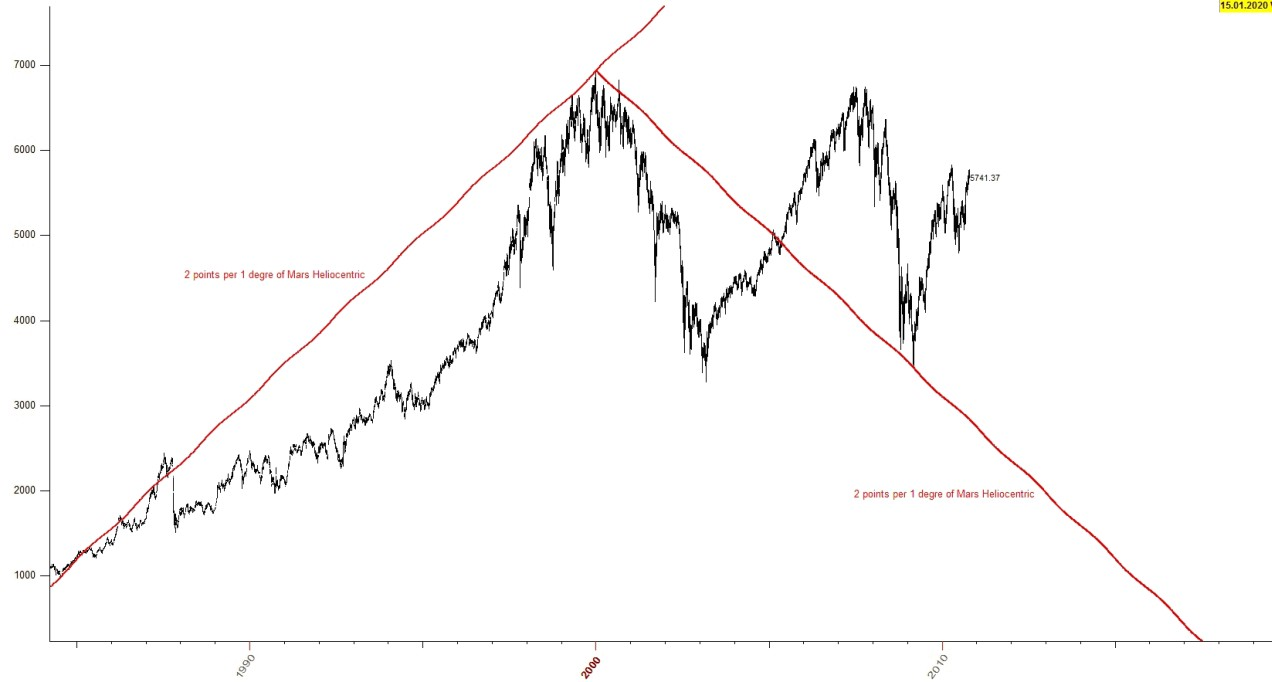Again ,a Mars Heliocentric planetary fan set at 2 point per 1 degree of Mars travelled ,from the October 15th 2007 Top ,going down

Hits the
April  16th 2010 Top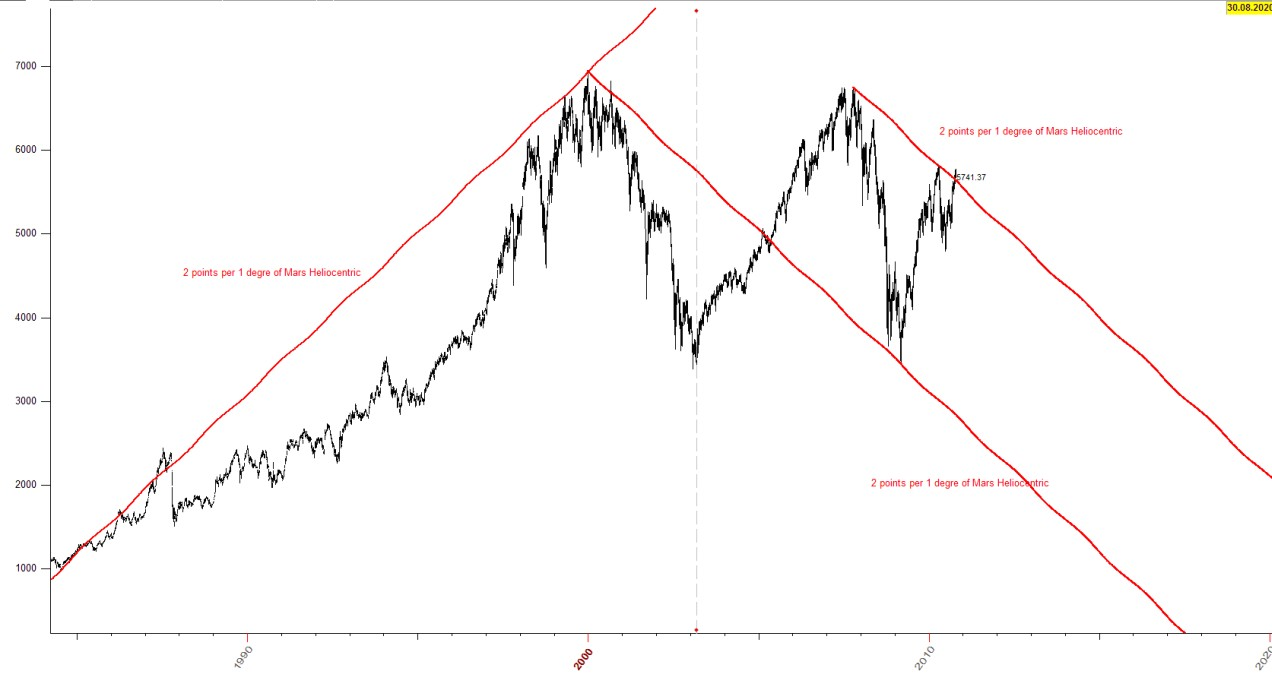From Dec 30 1999 Top to March 13th 2003 LOW ,  earth travelled 1153 degrees

And
Mars (Heliocentric ),travelled the same degrees from the March 12th 2003 LOWto the March 9th 2009 LOW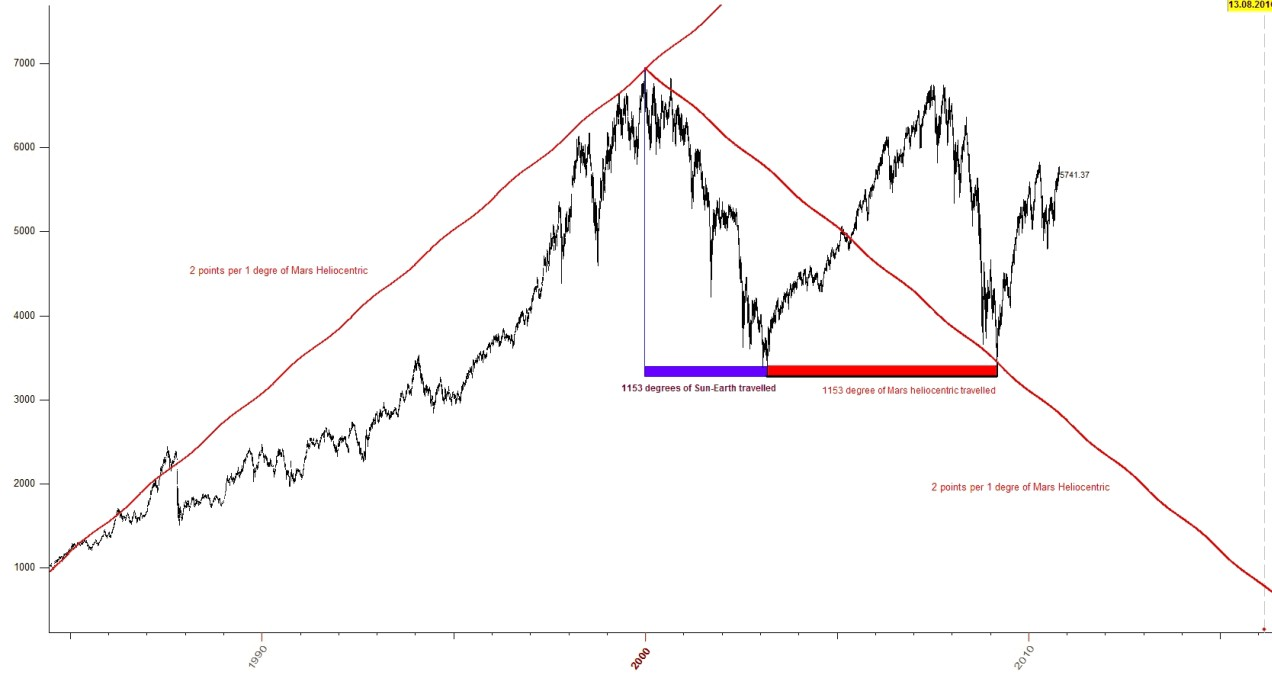Now using a Jupiter Heliocentric Fan ,set at various points per Jupiter degrees travelled:

From the
LOW of July 12th 1984 ,going upwards1984 LOW -1999 TOP ,was set to 12.5 points per 1 degree of Jupiter(Helio)
1984 LOW -2009 LOW ,was set to 3.33333 points per 1 degree of Jupiter (Helio) or 12/ 3.75 =3.333
1984 LOW -2003 LOW ,was set to 4  points  per 1 degree of Jupiter Heliocentric

Now:

The
Jupiter planetary fan set at 8.25 points per 1 degree gives the July 13th 2007 TOP

8.25 is   :   12.5 + 4  /  2 =8.25

1999 TOP   from 12.5 points per 1 degree ,and the LOW of 2003 , from  4 points per 1 degree

If we had the fan set a the “middle –point” of  12.5 and 4 ,in other words at 8.25 we would get the 2007 TOP

And if we used the middle point from the 1984-2003(4)  and 1984-2007(8.25) ,   8.25 + 4 /2 =6.125  we would get the April 16th
2010
TOP

From the LOW of October 8th 1998 if we use a SUN planetary fan  ,set at 1 degree  equal to 0.3 points ,both upwards and downards
from that point we get that: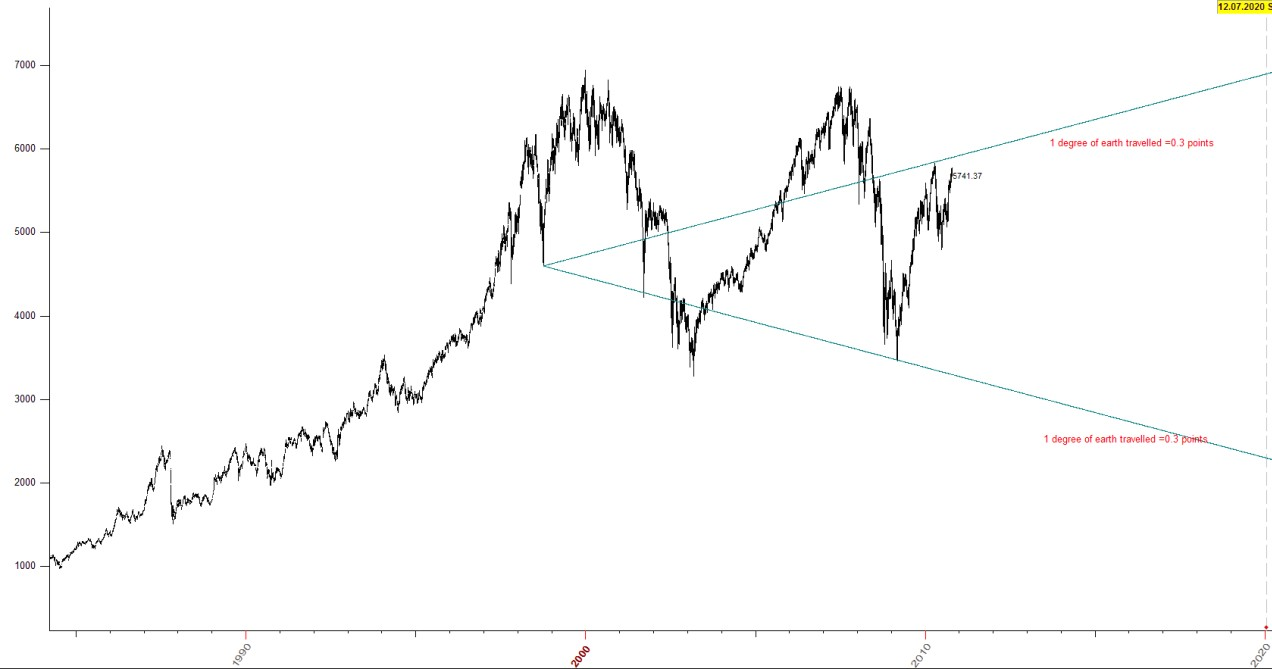April 16th 2010 TOP hit and LOW of March 9th 2009 hit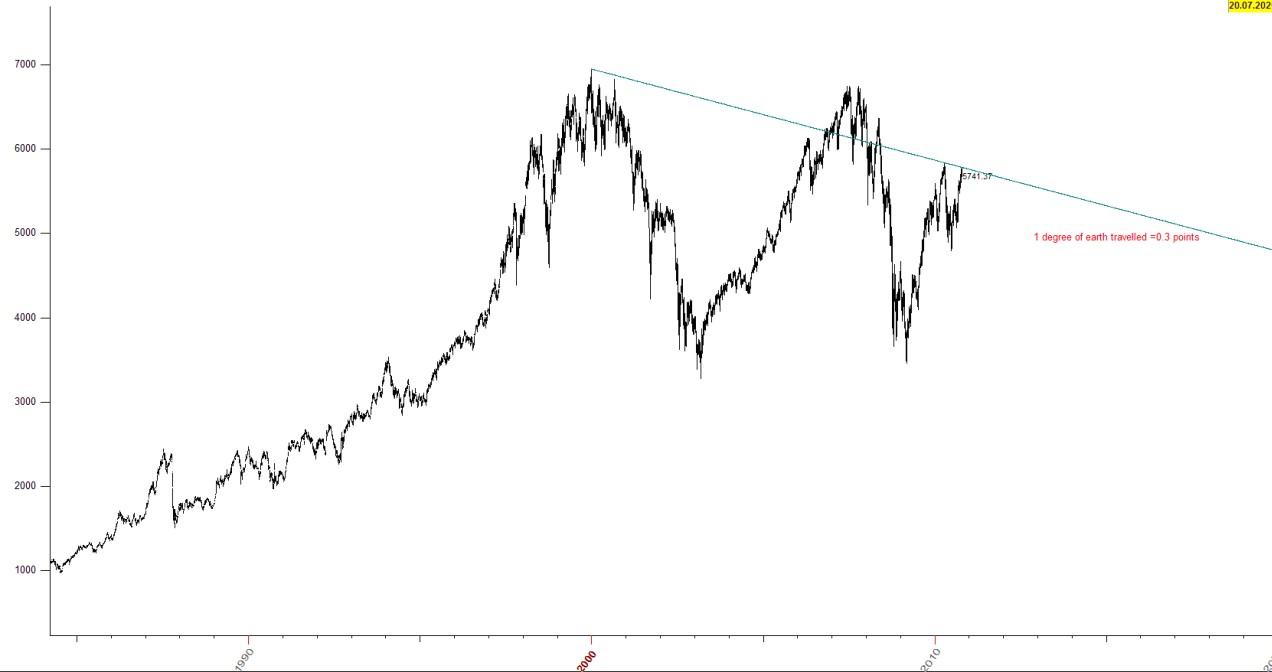Again : an Earth planetary fan set at 1 degree equal to 0.3 points ,going downwards ,from the Dec 30th 1999 High

Hits the April 16th 2010 High

Below we set an earth planetary fan at 1 degree equal to one point

Hits the
April 16th 2010 High from the 2003 LOW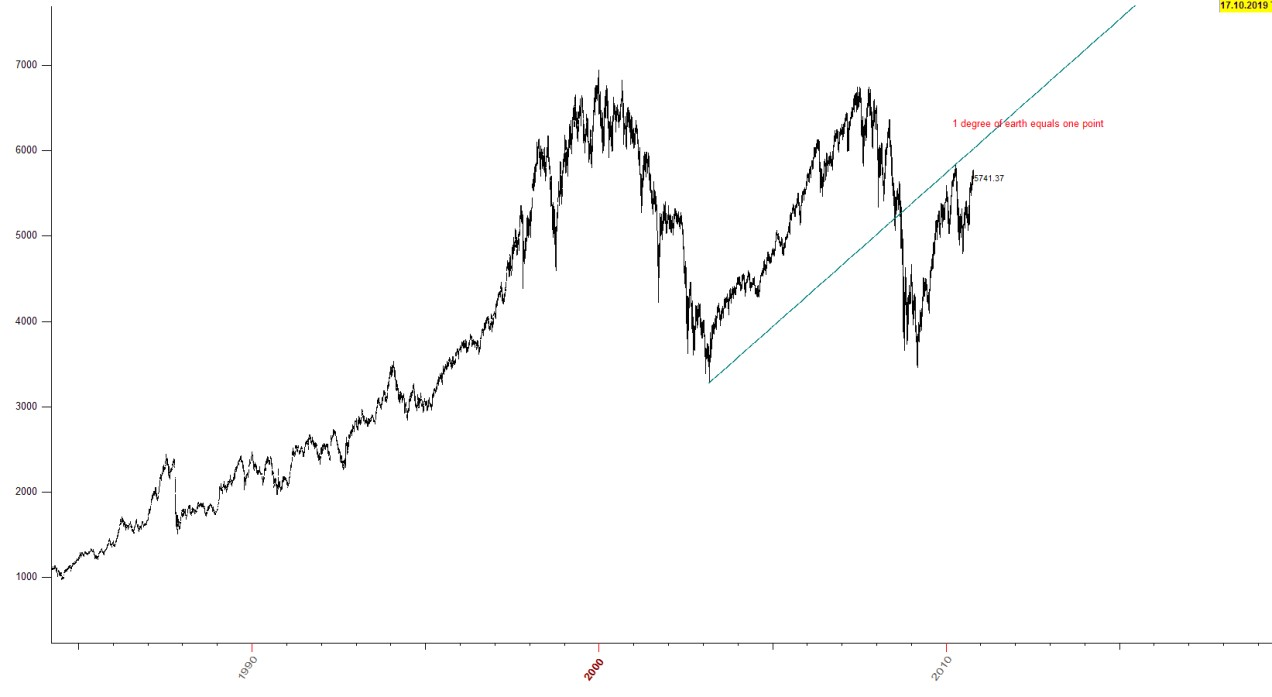All together now :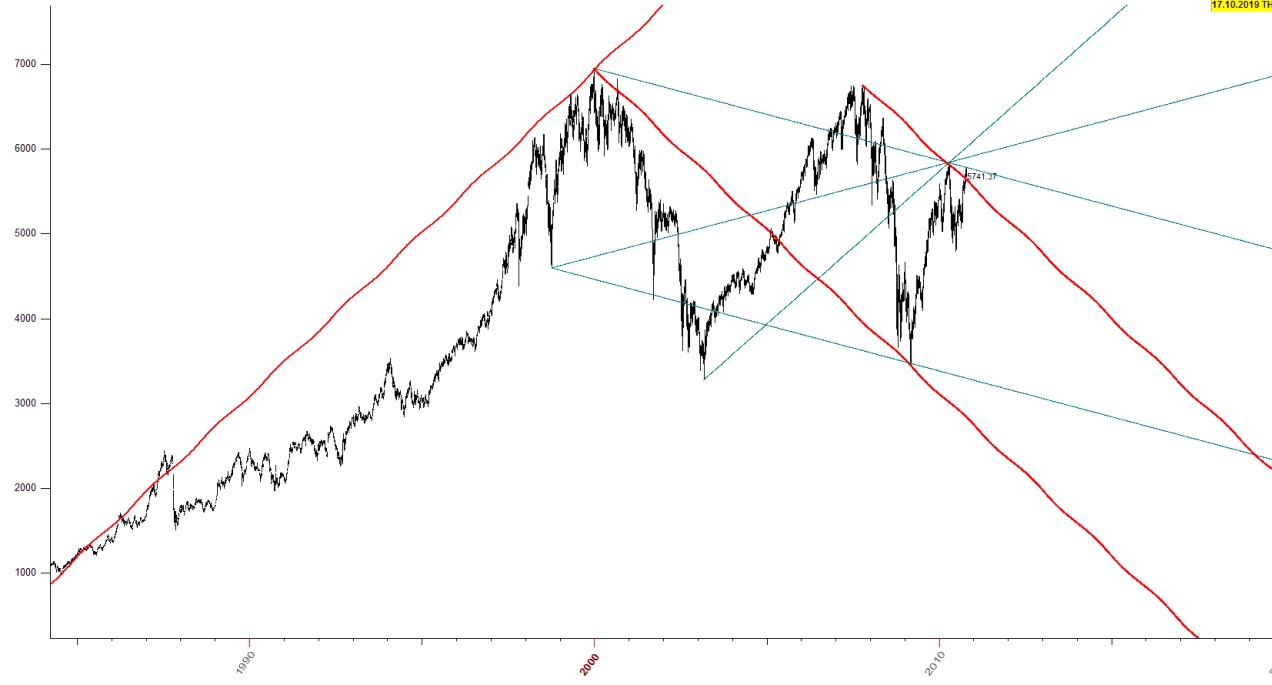I see a channelThis one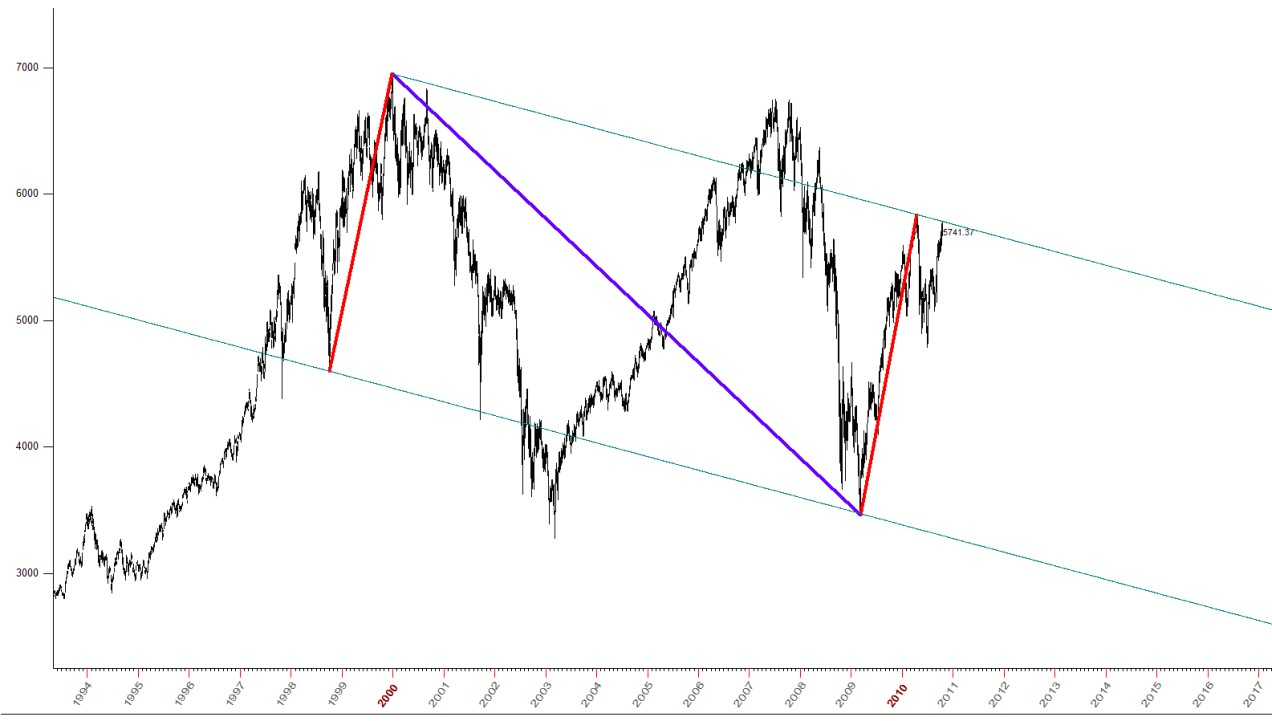The red lines are Sun_earth vectors

They are equal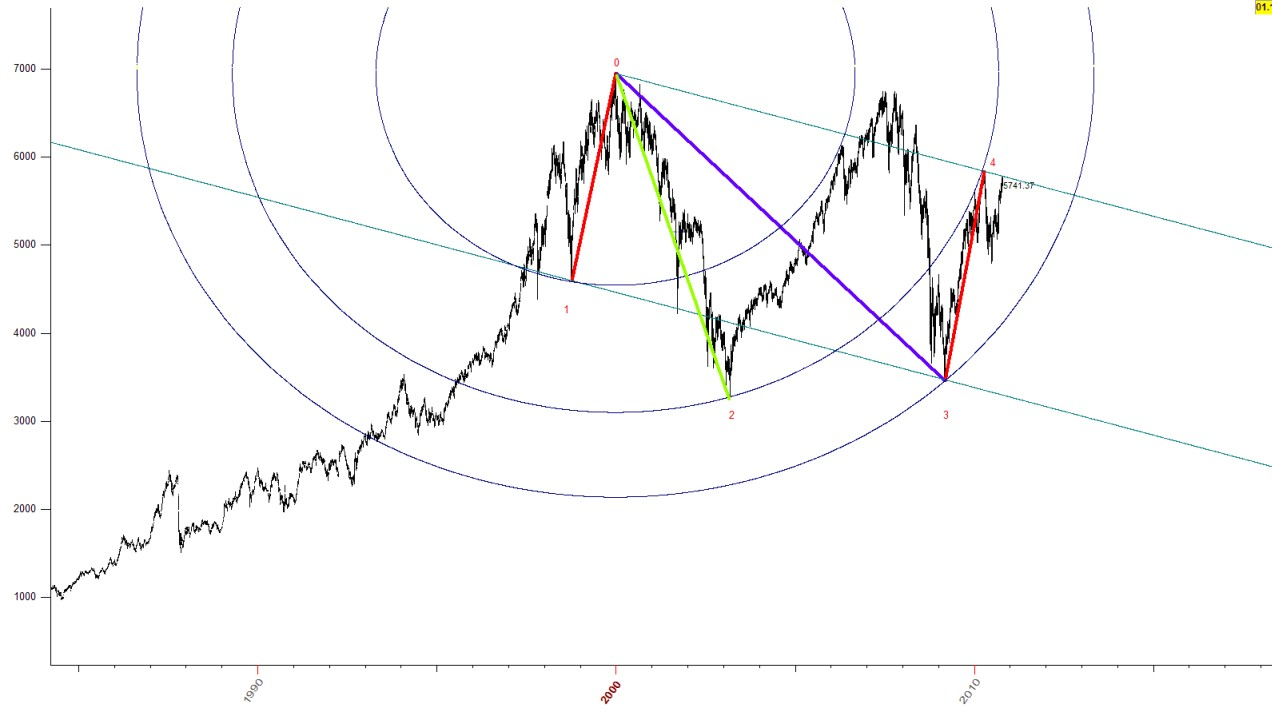Ill explain:

A planetary vector is :

The price range between two prices raised to the second power ,plus the degrees of the planet travelled raised to the second power ,and
then this sum raised at the ½ power

Or better said ,we take the square root of this sum

So the red first Vector ,from the
October 8th 1998 Low to the Dec 30 1999 High is calculated as follows:

4599.2 the low
6950.6 the high

The range   =  6950.6 -4599.2 =2351.4

Earth travelled from
October 1998 to December 1999, 443.32 degrees

So our vector is equal to :

(2351.4^2 + 443.32 ^2 ) ^ .5=2392.8256

The same red vector from the
2009 low to the April 2010 high

3460.7 the low
5833.73 the high

Th erange   2373.03

Earth travelled:

397.34 degrees

(2373.03 ^ 2 + 397.34 ^ 2 )^.5 =2406.065348

There is a difference between them but it is acceptable as it is

0.55% different

So 1 to 0 is equal to  3 to 4

An interesting geometry unfolds though if we use these ratios with our planetary vectors:

Lets do this:

The 0-2 vector(
green) is again an earth based vector (1 degree equals one point.We use the pythagorean theorem again to find its value)

So lets call our 0-1 vector 100% or 1 (BASE)

The 0-2 vector is   1.6 times bigger than the 0-1.

Or   0-1=(0-2) x .625

Or  the 0-1 is 62.5% of the 0-2 vector

Same is the (3-4) vector to the 0-2 vector

The
bluish vector 0-3 is  1.25 of 0-2
Or 125%

So we have:

0-1        62.5 %
0-2        100%
0-3        125%
3-4        62.5%

Green 0-2 beeing the base

But also 0-4 is equal to 0-2
Below I have inserted some other connections between vectors found in FTSE

I will leave it to you to make the connections

After all ,why should I have all the fun .RIght ?
red-red same
gree-green same

bluish' 1.4142 of reds

bluish twice as big as greens

red is  100%
green is 70.7% of red (.707 of 1 that is )     0.707= 1 /(square root of 2)

and
bluish is 141.42% to red or 1.4142 times bigger
violet equal vectors here

always  sun-earth based.

they are the same.  3848

middle circle is 1(100%)
inner is .707  (70.7%)
outer is 1.414 (141.42%)
first red vector  comes  from :

1987 low to 1996 low

10C199  stand for 10x360+199=3799   (  c=cycles)

second
red  vector (it is equal with the first)  comes  from 2003 low to 2007 high 10c200(1 off !)

then the
green is :

1996 low to 1999 high   9c322
and 1.4142 of that
green ( 9c322 x 1.4142)    from the 1996 low to 2007 high  and backwards to 1984 low
fisrt  green vector ,from 1984 low to 1994 low is 11c81 (4041)

then second
green vector ,from  low 1998 to high 1994 is half of it (50% or .5) of first green vector

you can see also 1984-1994 (100% ) and 1984 to 2010(July 1st ) 250% or 2.5 of 11c81
low 1992 to 1999 high red is 100% (14c344= 5384)

.707 of it is the
green from 1992 to September 21st 2001

then the two other greens are the same (all 3 are the same basically)

from
2003 low to 1999 high   0.707 x 14c344
from
2003 low to 2007 high   .707x 14c344

3 violets the same
2
greens the same

greens/violets =1.4142 / 1
greens same   (6c 244) =2404

blue  1.61803 of greens   1.618 x 2404=3889
 Some planetary fans below
Catch you laterThanks for dropping byPeter Palaskas